# 生物统计学与R极简手册

## 描述性统计量

### 计算描述性统计量

xxxxxxxxxxsummary(iris)​#查看常用的描述统计量
xxxxxxxxxx Sepal.Length    Sepal.Width     Petal.Length    Petal.Width          Species Min.   :4.300   Min.   :2.000   Min.   :1.000   Min.   :0.100   setosa    :50 1st Qu.:5.100   1st Qu.:2.800   1st Qu.:1.600   1st Qu.:0.300   versicolor:50 Median :5.800   Median :3.000   Median :4.350   Median :1.300   virginica :50 Mean   :5.843   Mean   :3.057   Mean   :3.758   Mean   :1.199 3rd Qu.:6.400   3rd Qu.:3.300   3rd Qu.:5.100   3rd Qu.:1.800 Max.   :7.900   Max.   :4.400   Max.   :6.900   Max.   :2.500​

### 形象化展示

xxxxxxxxxxboxplot(iris$Sepal.Length)# 使用箱线图展示某一列数据的分布情况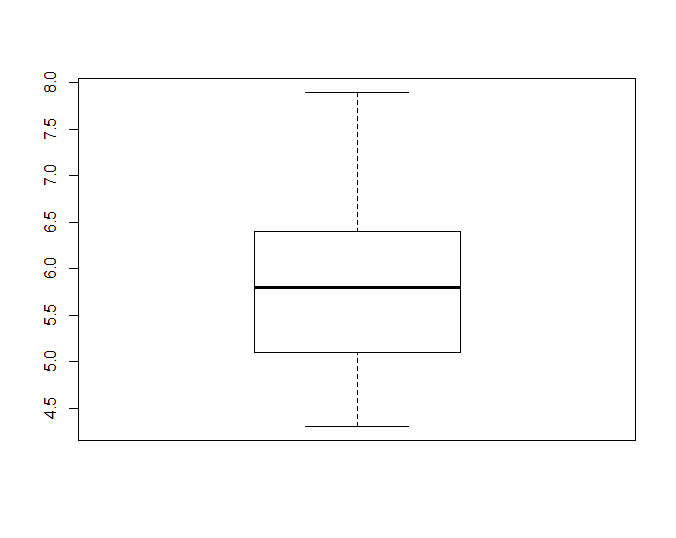xxxxxxxxxxhist(iris$Sepal.Length)# 使用直方图展示某一列数据的分布情况​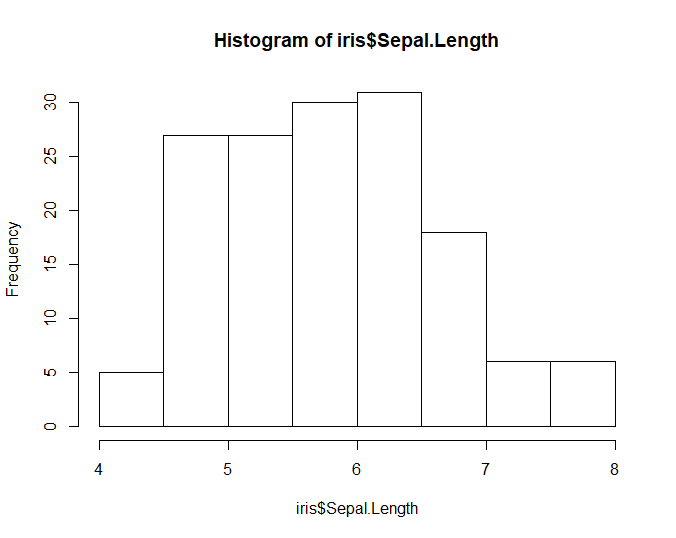xxxxxxxxxxplot(ecdf(iris$Sepal.Length))# 绘制简单的累积分布函数图展示某一列数据分布情况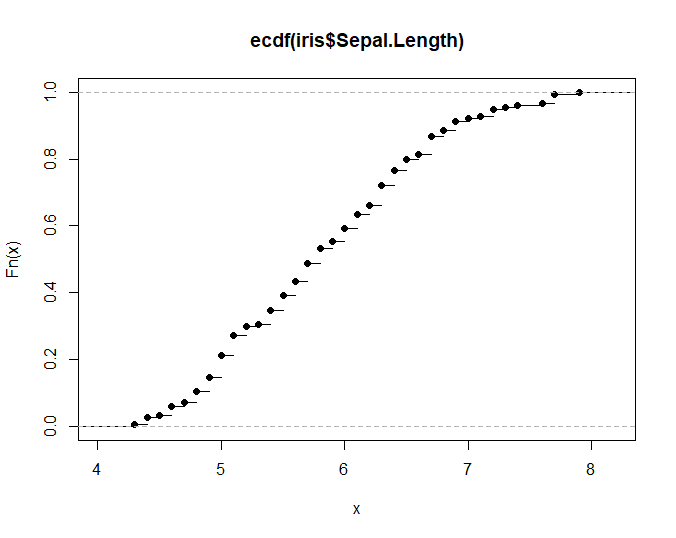## 概率相关内容 统计学中大量内容源于概率，学习统计学也就必须要了解一些概率中的基本概念，其中尤为重要的是条件概率，以及延伸出的贝叶斯定理（也许是最牛也是最难充分掌握的内容）。 ### 几个概念 样本空间(sample space)是所有可能结果的一个集合，事件(event)则是样本空间中所有感兴趣结果的一个子集。某事件的概率(probability)是该事件在无限次试验次数中的相对频率。 事件的交集并集和补集，概率的加法法则和乘法法则在本章中不再做介绍。 条件概率(Conditional Probability)用来描述与其他事件的发生相关的某事件的概率，通常被描述成“在A事件下发生事件B的概率”。在书写时用|表示，例如P(A|B)是在B发生的情况下A发生的概率。计算方式为AB同时发生的次数除以所有B发生的次数。 条件概率计算方法：$P(A|B)=P(A\cap B)/P(B)$ 可推出: $P(B|A)=P(A\cap B)/P(A)$ => $P(A\cap B)=P(A)\times P(B|A)$ 全概率公式：$P(B)=P(A)\times P(B|A)+P(A')\times P(B|A')$ （贝叶斯定理分母部分） 贝叶斯定理：$P(A|B)=\frac{P(A)\times P(B/A)}{P(A)\times P(B|A)+P(A')\times P(B|A')}$ 得到的贝叶斯定理可以帮助我们计算逆条件概率。 在实际的生物学数据处理的过程中，我们还会接触到灵敏度(sensitivity)特异度(specificity)假阳性(false negative)假阴性(false positive) 几个概念。在疾病相关研究中，对于某一个症状，灵敏度指发病后出现症状的概率，特异度是不发病时不出现症状的概率。假阳性是指实验结果阳性但是实际为阴性，假阴性是指实验结果为阴性但是实际为阳性。基于灵敏度和特异度可以使用ROC曲线，通常来说在两个检验中，曲线下面积大的较好。 概率能够告诉我们事件发生的可能性，但如果想要利用概率预测未来的结果并且评估预测的确定性就需要引入概率分布。 ### 离散概率分布 随机变量： 样本空间中，对不同事件指定有相应概率的数值函数。 随机变量是可以等于一系列数值的变量，这些值都和一个特定概率关联。它的写法是$P(X=x)$，表示随机变量X取特定值为x时的概率。随机变量包括离散和连续两种形式，所谓离散指变量只能取一些确定值。连续指的是有无限多种可能取值。 概率分布也叫概率质量分布，$P(X=x)$。在描述统计量中，样本的频数分布描述每个取值及对应发生次数，如果样本总数除以对应发生次数，得到的频率分布就类似于这里的概率分布。后面会提到的“拟合优度检验”就是比较有限样本频率分布和概率分布的差异。 如果将随机变量和样本对应起来理解，样本中均值的概念在总体（随机变量）中称为期望，也叫作总体均值，表示为$\mu$（和均值一致）或者$E(X)$。计算方式是将每个可能值和概率相乘再把所有乘积相加。和均值类似，这里的期望也无法描述相关数值分散程度。 同样，在随机变量中也有类似于样本方差的概念，称为总体方差（随机变量方差），用来表示分散程度。其计算公式为 $Var(X) = E(X-\mu)^{2}$。而概率分布的标准差$\sigma$同样是方差的平方根。 计算$E(X-\mu)^{2}$时，首先计算每个数值x的$(x-\mu)^{2}$,然后再将结果乘以概率，最后把所有结果相加。 在数据集中，方差和标准差表示的是数据和均值的距离，在概率分布中表示特定数值概率的分散情况。方差越小，结果越接近期望。 累加分布函数(cumulative-distribution function, cdf): 随机变量X，对于X的任一指定值x，概率值$P(X\leq x)$。即随机变量取值不大于指定值的概率。记作$F(x)$ ### 常见的离散概率分布 几何分布：进行一组相互独立实验，每次实验有成功失败两种可能且每次试验概率相等，想知道第一次成功需要进行的试验次数。 $P(X=r)=pq^{r-1}$ $P(X>r)=q^{r}$ $P(X \leq r)=1-q^{r}$ 期望$E(X)=\frac{1}{x}$；方差 $Var(X)=\frac{q}{p^{2}}$ 二项分布：进行一组相互独立试验，每次实验有成功失败两种可能，每次试验概率相等且试验次数有限，想知道在有限次试验中成功的次数。 $P(X=r)=C^{r}_{n}p^{r}q^{n-r}$ 期望$E(X)=np$；方差$Var(X)=npq$ 二项分布和几何分布差别在于，前者试验次数固定求成功概率，后者求第一次成功前试验次数。 泊松分布：常与稀有事件相关，单独事件在给定区间（区间可以是时间或者空间）内随机独立发生，该区间内的事件平均发生次数已知且为有限值。这个值用$\lambda$表示。给定区间内发生r次事件的概率计算公式：$P(X=r)=\frac{e^{-\lambda}\lambda^{r}}{r!}$ 期望是给定区间内能够期望的事件发生次数λ，方差也是λ。如果一个离散随机变量的一批数据计算后方差和均值近似相等，则可以推测样本符合泊松分布。 当二项分布的p很小且n非常大时，$npq\approx np$,方差和期望近似相等，可以用泊松分布来近似二项分布，从而使计算简化。通常，n大于50且p<0.1时为典型的近似情况。 ### 连续概率分布 当数据连续分布，人们关心的是取得某一个特定范围的概率。 概率密度函数(probability density function, pdf):本质是一个函数，用这个函数可以求出在一个范围内某连续变量的概率，同时该函数可以指出该概率分布的形状。换句话讲，任意a,b两点之间及函数对应曲线下组成的面积等于随机变量X落在ab间的概率。曲线下面积总和是1。 概率密度可以指出各种范围内的概率大小，用面积来表示。概率密度只是表示概率的一种方法而非概率本身。 累加分布函数a点上的值等于随机变量X取值$\leq a$的概率，也是概率密度函数a左边曲线下的面积。 正态分布是连续数据的一种理想状态。正态分布的概率密度函数是一条对称的钟形曲线，均值具有最大的概率密度，偏离均值概率密度逐渐变小。参数$\mu$是均值，也是曲线的中央位置，$\sigma$表述曲线的“胖瘦”。 连续随机变量X符合均值为$\mu$，标准差为$\sigma$的正态分布时写作$X\sim N(\mu,\sigma^{2})$. 线性变换：$aX+b \sim N(a\mu +b,a^{2}\sigma^{2})$ 当X和Y相互独立（彼此之间没有影响）时： $X+Y \sim N(\mu_{x}+\mu_{y},\sigma_{x}^{2}+\sigma_{y}^{2})$ $X-Y \sim N(\mu_{x}-\mu_{y},\sigma_{x}^{2}+\sigma_{y}^{2})$ 期望$E(X_{1}+X_{2}+...+X_{n})=nE(X)$；方差$Var(X_{1}+X_{2}+...+X_{n})=nVar(X)$ 当X和Y并不彼此独立时，使用协方差(Covariance)来描述两个随机变量间的关系，记做Cov(X,Y) $Cov(X,Y)=E[(X-\mu_{x})(Y-\mu_{y})]$ 二项分布正态近似：通常情况，当二项分布满足$np\geq 5$ (也有建议$npq\geq 5$)时，可以用正态分布代替二项分布。其中$\mu = np$,$\sigma^{2}=npq$。另外，当泊松分布的λ>15时，也可以用正态分布进行近似。 ## 估计 在通常的试验中我们获得的信息总是来自样本，想要知道总体的参数，只能通过已有样本参数进行估计。 样本均值是总体均值的点估计，通常样本均值用$\overline x$表示，总体均值用$\mu$表示。 在估计总体方差$\sigma^2$时，计算公式为$\sigma^2=\frac{\Sigma(x-\overline x)^2}{n-1}$ 用样本方差估计总体方差会使得估计结果偏低，样本越小两个方差的差别可能就越大。在这里，估计总体方差的公式中除的是$n-1$，能够更接近总体方差。另外，总体方差点估计公式通常记做$s^2$,写作：$s^2=\frac{\Sigma(x-\overline x)^2}{n-1}$ 用样本均值估计总体均值时也会产生误差，均值的标准误差是$\sigma/\sqrt{n}$，估计量是$s/\sqrt{n}$。标准误差表示了样本均值的分散情况，从公式中我们可以看出，n越大，用样本均值估计总体均值越准确。当样本足够大（大于30），即便总体不符合正态分布，但从中取出的样本均值分布仍然近似于正态分布（中心极限定理）。$\overline X \sim N(\mu, \sigma^2/n)$ 除了对总体进行点估计以外，我们往往还会对总体进行区间估计，即对通过点估计得到的结果加减一定范围的误差。 ## 相关性分析 本节提到的相关性分析和后面会提到的t-test, ANOVA 以及回归分析等被称为参数检验，这些检验在进行时我们常默认数据符合一定前提条件，如符合正态分布和方差相等等。当样本数量大于30时，根据中心极限定理，我们通常认为数据符合正态分布；在进行t-test 和ANOVA 分析时，还需要满足样本方差相等。 在进行各种检验之前需要初步检验数据是否符合某种检验的前提条件，如果不符合则应该考虑使用非参数检验或其他方法。 ### 正态分布评估 在评估数据集是否符合正态分布时通常会采用Shapiro-Wilk’s test图示(Q-Q plot)结合的方法。使用Q-Q plot(quantile-quantile plot)的结果较直观，使用Shapiro-Wilk’s test显著性检验的方法更准确（相对而言）。 Shapiro-Wilk’s test 结果受样本量的影响非常大，当样本量很大时即便数据符合正态分布也容易出现p值很小进而拒绝原假设的情况（该检验原假设是样本来自于正态分布）。样本量很小时即便真实数据不s是来自正态分布，也可能接受原假设。 这里试举一例 xxxxxxxxxx# 分别随机生成两组二项分布和指数分布随机数set.seed(90)x <- rbinom(15,8,0.7)y <- rexp(15,0.5)shapiro.test(x)shapiro.test(y)​# Shapiro-Wilk normality test## data: x# W = 0.95996, p-value = 0.6917### Shapiro-Wilk normality test## data: y# W = 0.96168, p-value = 0.7216 可以发现，即便我们生成的两个样本都不是正态分布，但是检验的结果仍然没有拒绝原假设（没有拒绝不等于接受原假设）。好在R中该函数限制检测的样本个数是3到5000。因此，同时结合图像来分析还是很必要的。 一般使用Q-Q plot来检验是否符合正态分布，R中默认的函数是qqnorm()；ggplot2中可以使用函数qplot()；qqpubr包是基于ggplot2二次开发的简易升级版，操作更加友好，可以使用函数ggqqplot() 下面利用生成的数据绘图。 xxxxxxxxxx# 生成符合正态分布的一组数据并绘图z <- rnorm(50)qqnorm(z)​library(ggplot2)qplot(sample=z)​library(ggpubr)ggqqplot(z)​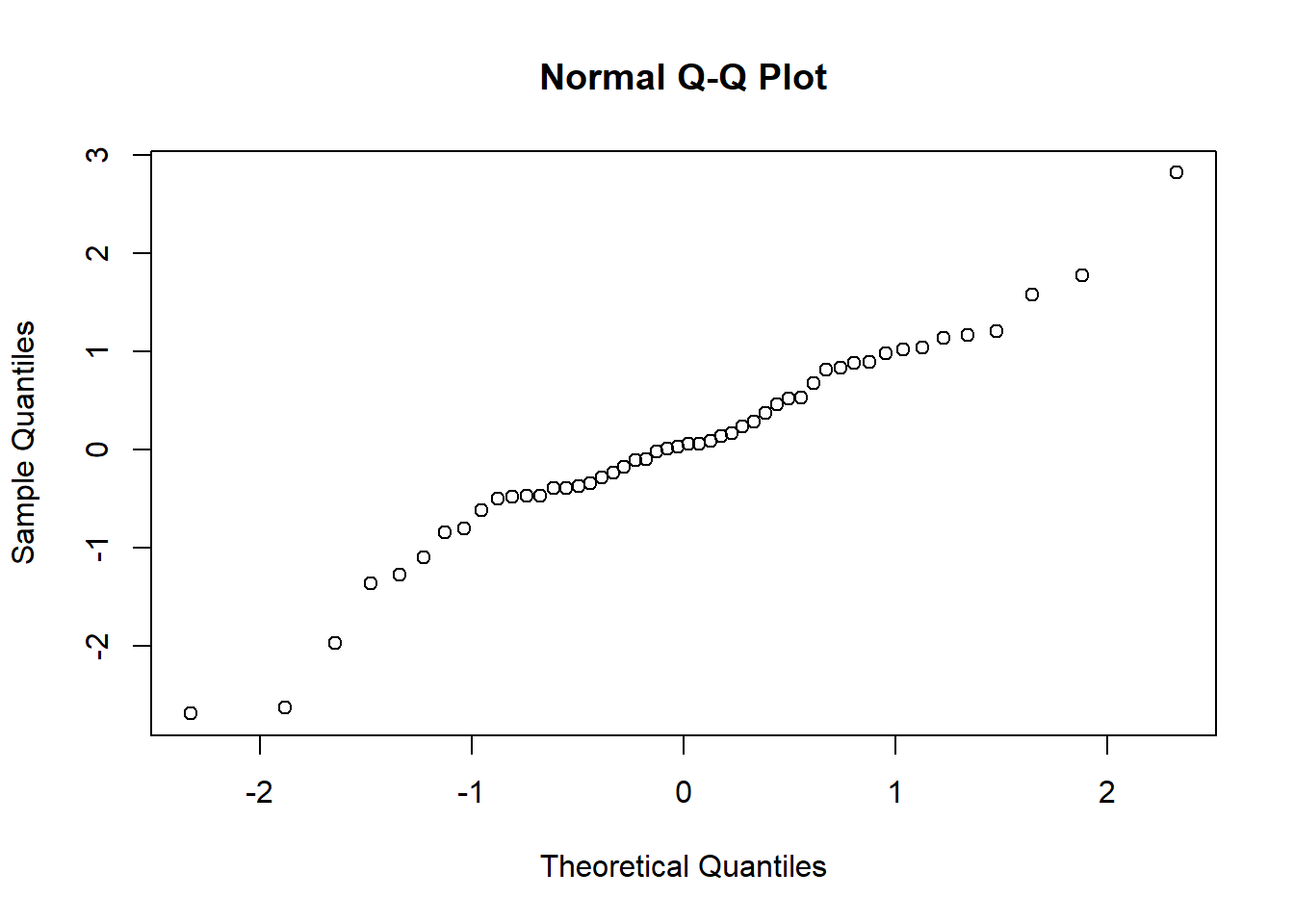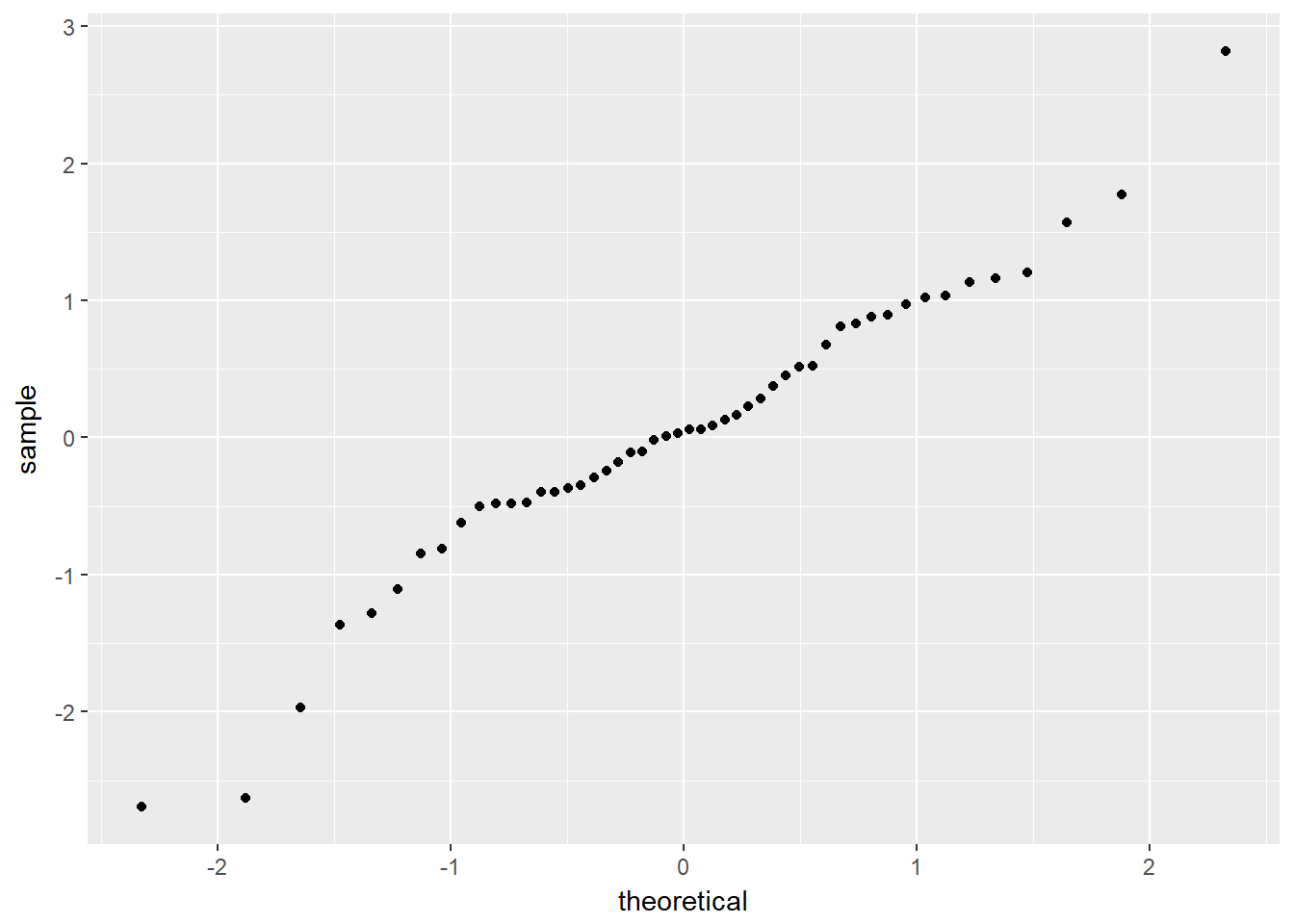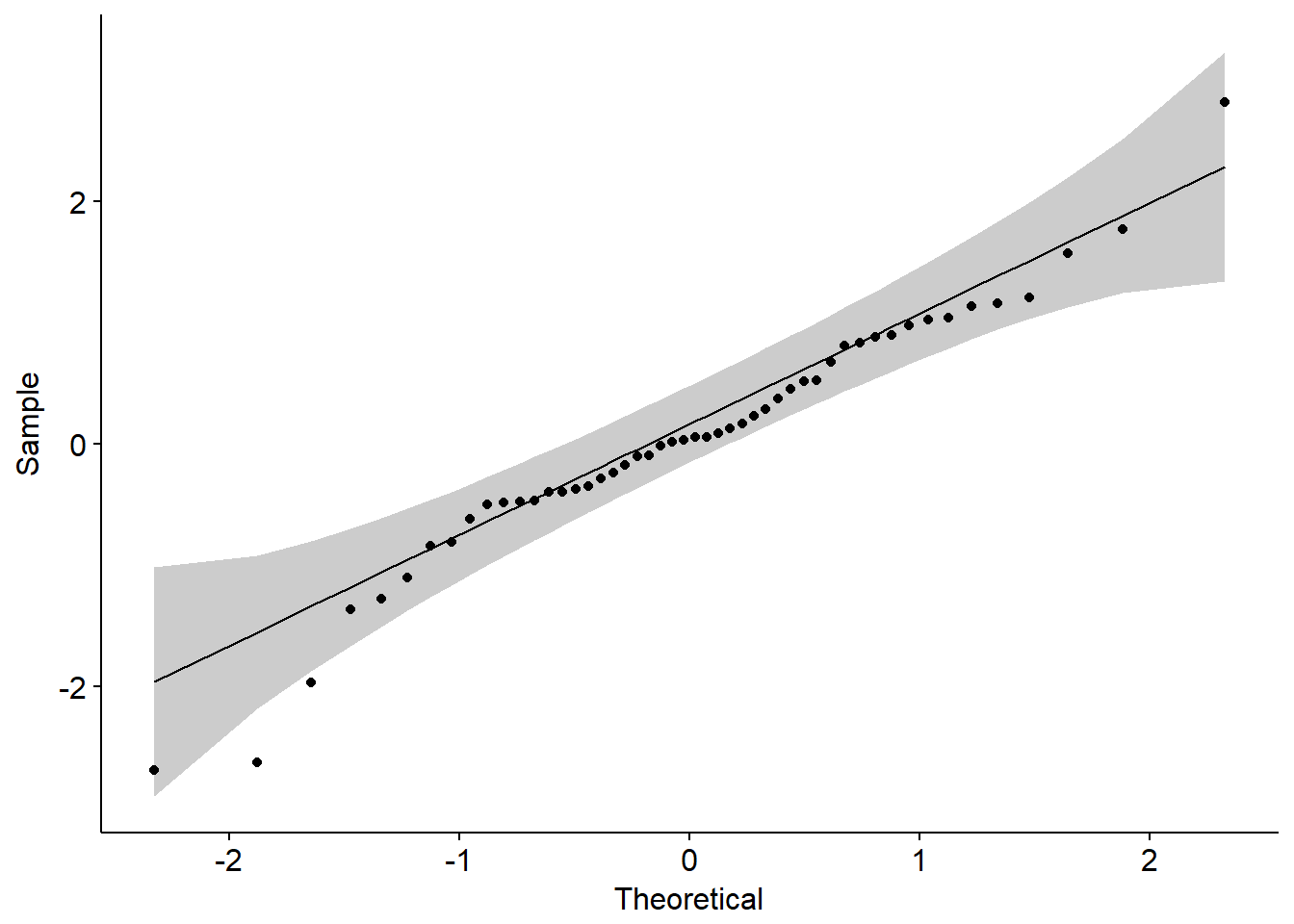### 相关性分析 Pearson相关系数、Spearman相关系数、Kendall相关系数都可以用来表示变量之间的相关性，一般情况使用pearson相关系数更多，如果明确样本不符合正态分布可以使用kendall或者spearman相关系数。这三种相关系数都可以通过cor()函数来进行计算，下面通过R已有数据集cars，查看汽车车速和刹车距离之间的相关性。 pearson相关系数计算公式 $r = \frac{\sum{(x-m_x)(y-m_y)}}{\sqrt{\sum{(x-m_x)^2}\sum{(y-m_y)^2}}}$ 其中m表示均值。 xxxxxxxxxxcor(cars, method = "pearson")​ ### 相关性可视化展示 可以使用散点图进行两个变量之间的相关性展示。 xxxxxxxxxxplot(cars)​ggplot(cars, aes(x=speed, y=dist))+ geom_point()​ggscatter(cars,x="speed", y="dist",\add = "reg.line", conf.int = T,cor.coef = T)​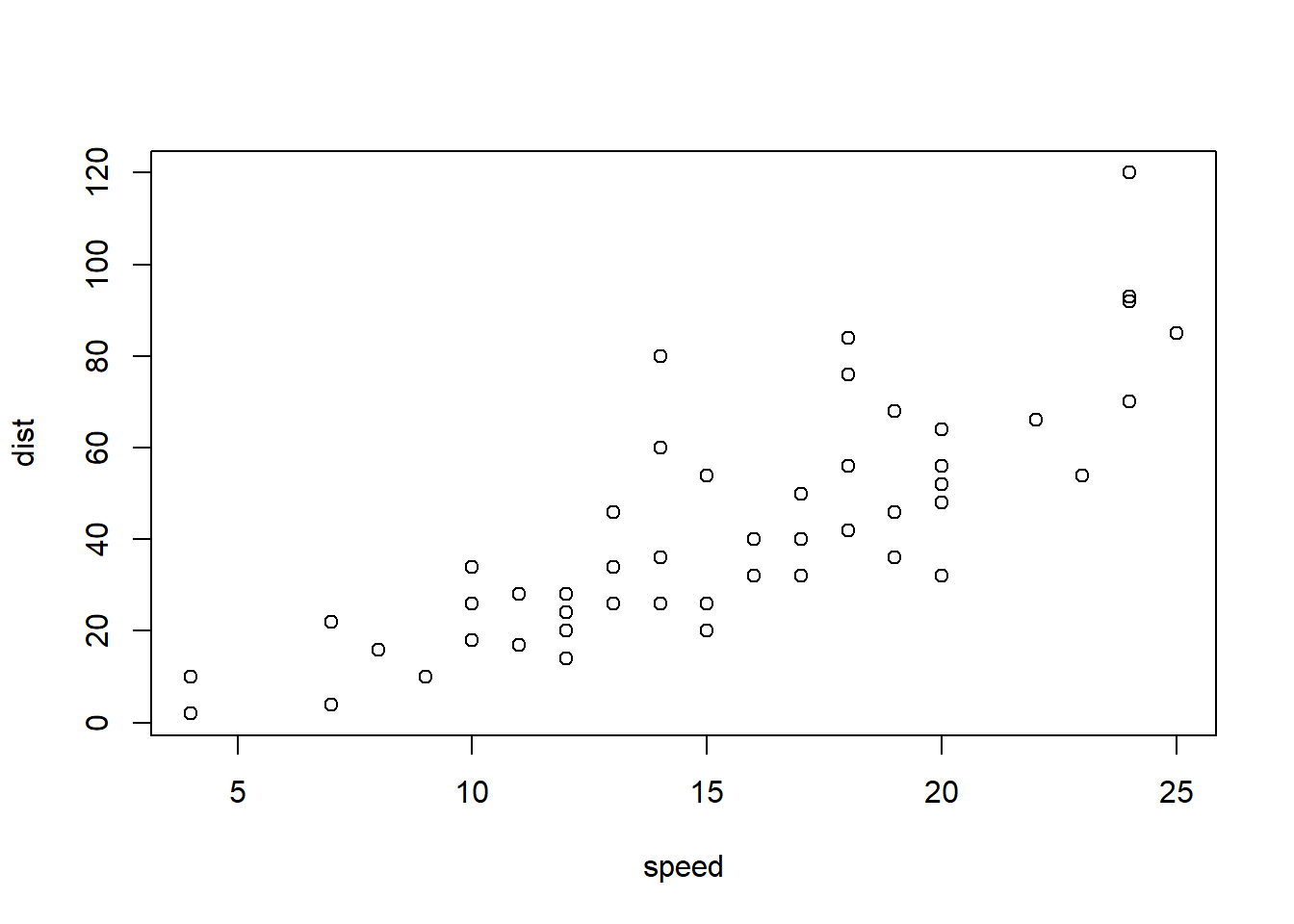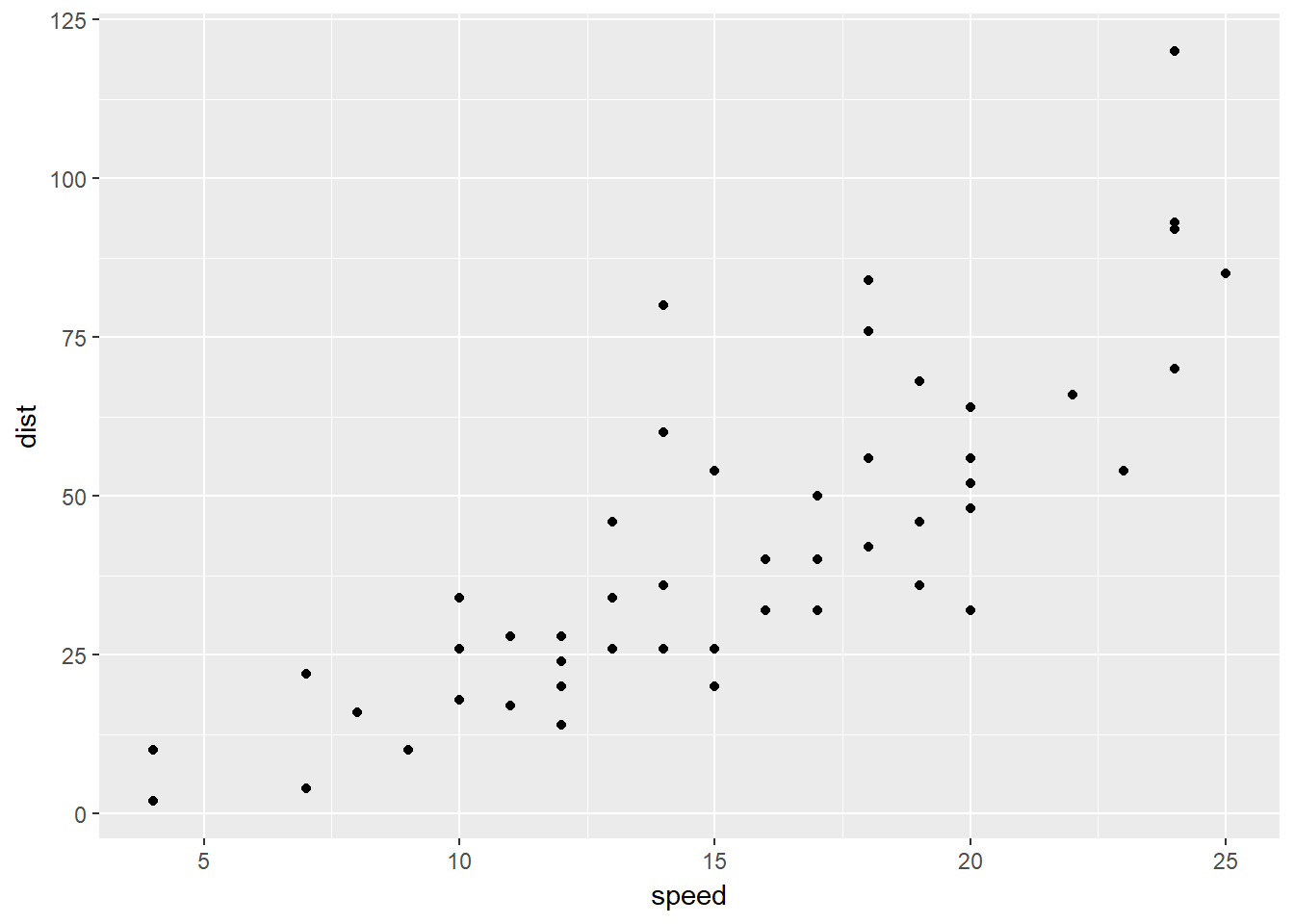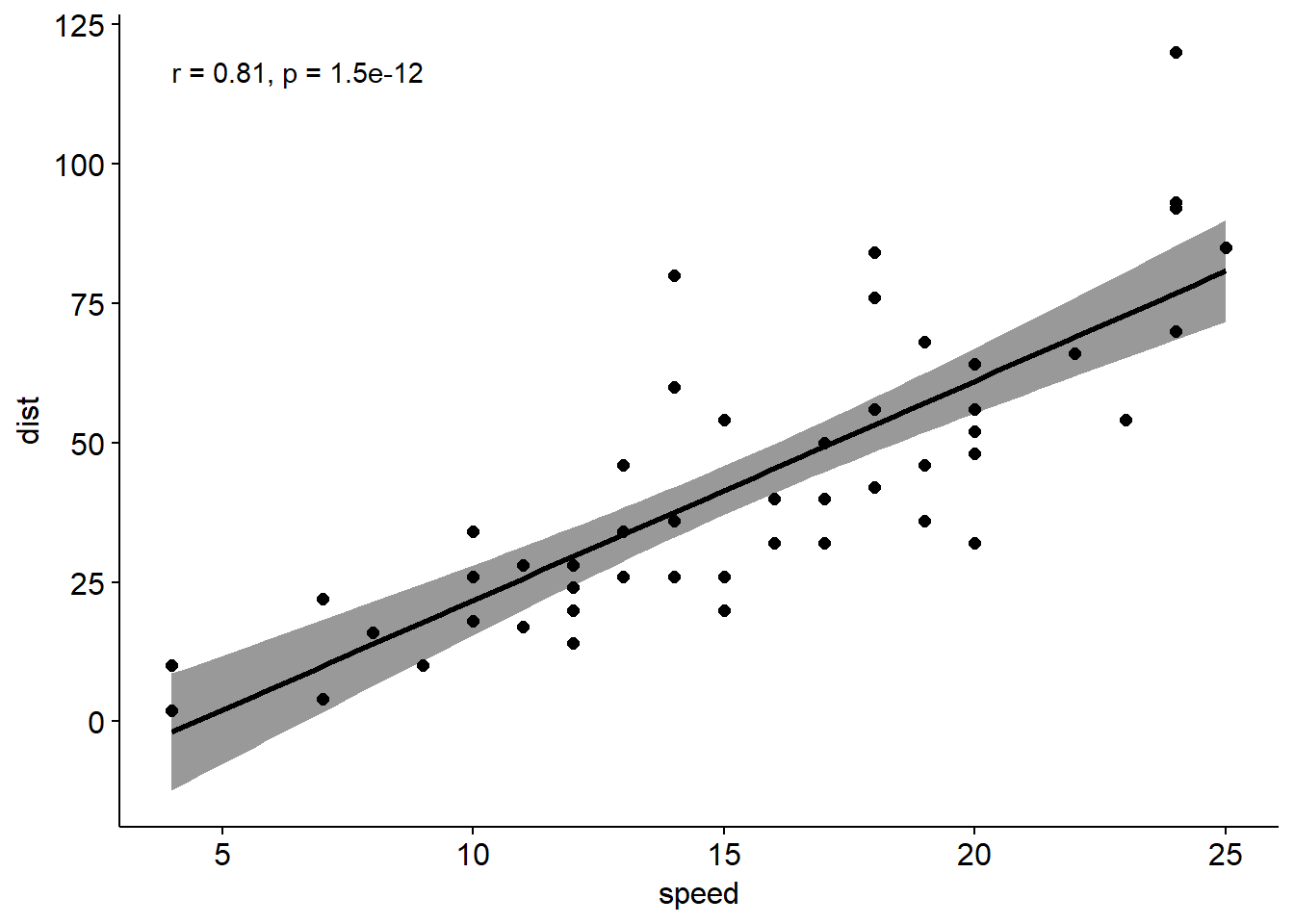### Pearson 相关性检验 前面我们只是计算了两个变量之间的相关性，还应该对相关进行显著性检验。原假设为变量之间没有相关性，使用函数为cor.test() xxxxxxxxxxcor.test(cars$speed,cars$dist,\alternative = "two.side", method = "pearson")​ 统计结果中，t表示t检验统计量，df表示自由度，pvalue是t检验的显著性水平，conf.int表示95%的置信区间，sample estimates 是相关系数。相关系数越接近-1表示负相关，接近1表示正相关。 ## 单双样本均值分析 根据数据组数的不同，均值比较可以分为单样本、双样本和多样本。本节首先介绍单样本和双样本。 ### 假设检验一般步骤 谈具体的假设检验之前首先介绍假设检验的一般步骤。 1. 确定假设 原假设(null hypothesis, $H_0$) 指需要检验的假设，只要当我们有足够的证据时才能否定。在某种意义上与原假设相反的的假设被称为备择假设（alternative hypothesis，$H_1$）。 假设检验是无法给出绝对证明的，我们只能在假定原假设为真的情况下通过假设检验来判断结果是否可信。如果结果极不可能发生，则拒绝原假设，进而接受备择假设。 根据接受假设和真实情况之间的关系，会有四种可能发生 其中$H_0$为真但是被拒绝的错误概率称为I型错误，通常用$\alpha$表示，也称为显著性水平$H_1$为真但是接受$H_0$的错误概率称为II型错误，常用$\beta$表示。 检验的功效（power）=1-$\beta$。虽然我们希望$\alpha$$\beta$都可以尽可能小，但这两者往往是矛盾的，通常我们会先固定$\alpha$在某个水平，再找合适的检验使$\beta$尽可能小。 1. 选择检验统计量 检验统计量（test statistic）是用于对假设进行检验的统计量，进行检验的过程建立在这个统计量之上。 1. 决定拒绝域 拒绝域是可以不接受原假设的一组数值，其分界点被称为临界点。为了求出拒绝域首先要确定假设检验的显著性水平，也就是当样本结果的不可能发生程度多大时就可以拒绝原假设。 单尾检验指检验的拒绝域落在可能数据集的一侧（左侧或者右侧），双尾检验的拒绝域则分布在数据集的两侧，对于检验水平是$\alpha$的双尾检测而言，两侧分别是$\alpha/2$。如果备择假设的表示是“不等于”，则应该使用双尾检验。 1. 求出p值 p值取决于检验统计量和拒绝域。表示得到更加极端结果的概率。 1. 判断样本结果是否在拒绝域内 2. 做出决策 ### 单样本t-test 说完假设检验的基本流程之后，下面介绍和均值比较相关的假设检验，首先是单样本t-test 在数据符合正态分布的前提下使用单样本t-test来比较一组样本的均值和已知（理论/总体）均值，所谓的已知均值可能来自于之前的实验数据或者理论值。根据研究问题（原假设）的不同又分为双尾（不等）和单尾检验（大于或者小于）。 统计量计算公式为 $t=\frac{\overline{X}-\mu_0}{s/\sqrt{n}}$ 其中$\overline X$表示样本均值，n表示样本量，s是样本标准差（总体方差未知），$\mu_0$表示理论值。通过统计量t和自由度，我们可以计算出对应的p值。 使用R进行单样本t-test，这里借用R自带数据集PlantGrowth的10个对照组数据。 xxxxxxxxxxweight <- PlantGrowth$weight[PlantGrowth$group=="ctrl"]shapiro.test(weight)summary(weight)​# Shapiro-Wilk normality test##data: weight#W = 0.95668, p-value = 0.7475## Min. 1st Qu. Median Mean 3rd Qu. Max.# 4.170 4.550 5.155 5.032 5.293 6.110​ 可以发现均值大概是5左右，且数据符合正态分布。下面检验和理论值7相比的情况，原假设是等于7，备择假设是不等于7，所以采用双尾检验。 xxxxxxxxxxt.test(weight,mu = 7)​# One Sample t-test##data: weight#t = -10.673, df = 9, p-value = 2.075e-06#alternative hypothesis: true mean is not equal to 7#95 percent confidence interval:# 4.614882 5.449118#sample estimates:#mean of x# 5.032​ 通过上述结果看出统计量t为-10.673，自由度是9，p值是2.075e-06<0.05，所以拒绝原假设。 ### 单样本Wilcoxon 符号秩检验 如果样本数据没有通过正态分布检验就要采用单样本wilcoxon符号秩检验进行计算。使用该检验需要满足的条件是样本值均匀地分布在均值两侧。 其R中的函数为wilcox.test() xxxxxxxxxxwilcox.test(weight,mu=7) 通过结果可知拒绝原假设。 ### 独立双样本t-test 两个独立样本是指两个比较的样本之间没有关联，不互相影响。使用双样本独立t-test的前提是两个样本符合正态分布且方差相等 独立双样本t-test其统计量计算公式为 $t = \frac{\overline{X}_1 - \overline{X}_2}{\sqrt{ \frac{S^2}{n_1} + \frac{S^2}{n_2} }}$ $\overline{X}$分别表示两个样本的均值，n表示样本量。$s^2$表示两个独立样本的方差合并估计，计算公式为 $s^2 = \frac{(n_1-1)s^2_1+(n_2-1)s^2_2}{n_1+n_2-2}$ 依旧以PlantGrowth数据进行举例，PlantGrowth共有三组数据，在这里取两组进行分析。 xxxxxxxxxx# 查看各组基本信息library(dplyr)tmp1<-group_by(PlantGrowth,group) %>% summarise(count=n(),mean=mean(weight),sd=sd(weight))tmp1​# A tibble: 3 x 4# group count mean sd# <fctr> <int> <dbl> <dbl>#1 ctrl 10 5.032 0.5830914#2 trt1 10 4.661 0.7936757#3 trt2 10 5.526 0.4425733 进行独立双样本t-test前，首先验证数据是否符合正态分布(Shapiro-Wilk normality test)以及两个样本方差是否相等(F-test) 关于方差比较 • 两个方差比较通常使用F-test，在R中的函数为var.test()，进行F-test 检验前一定要首先进行正态分布检验，该统计量对于正态分布这个前提条件非常敏感； • 多个方差进行比较还可以使用Bartlett’s test，该方法同样要求满足正态分布前提条件； • 如果不能确定数据是否符合正态分布，可以使用Levene’s test 进行检验，其对数据正态分布的要求并非十分严格； • Fligner-Killeen test 则是一种非参数检验方法，数据可以不满足正态分布； • 关于方差比较部分内容，在本章不再展开。 xxxxxxxxxxshapiro.test(PlantGrowth$weight[PlantGrowth$group=="ctrl"])shapiro.test(PlantGrowth$weight[PlantGrowth$group=="trt1"])var.test(PlantGrowth$weight[PlantGrowth$group=="ctrl"], PlantGrowth$weight[PlantGrowth$group=="trt1"])​# Shapiro-Wilk normality test##data: PlantGrowth$weight[PlantGrowth$group == "ctrl"]#W = 0.95668, p-value = 0.7475### Shapiro-Wilk normality test##data: PlantGrowth$weight[PlantGrowth$group == "trt1"]#W = 0.93041, p-value = 0.4519### F test to compare two variances##data: PlantGrowth$weight[PlantGrowth$group == "ctrl"] and PlantGrowth$weight[PlantGrowth$group == "trt1"]#F = 0.53974, num df = 9, denom df = 9, p-value = 0.3719#alternative hypothesis: true ratio of variances is not equal to 1#95 percent confidence interval:# 0.1340645 2.1730025#sample estimates:#ratio of variances# 0.5397431 满足前提条件，继续方差相等的独立双样本t-test xxxxxxxxxxt.test(PlantGrowth$weight[PlantGrowth$group=="ctrl"], PlantGrowth$weight[PlantGrowth$group=="trt1"], var.equal = T )​# Two Sample t-test##data: PlantGrowth$weight[PlantGrowth$group == "ctrl"] and PlantGrowth$weight[PlantGrowth$group == "trt1"]#t = 1.1913, df = 18, p-value = 0.249#alternative hypothesis: true difference in means is not equal to 0#95 percent confidence interval:# -0.2833003 1.0253003#sample estimates:#mean of x mean of y# 5.032 4.661 ### 独立双样本Wilcoxon test 当两个样本不满足正态分布时，使用Wilcoxon秩和检验进行非参数检验。 xxxxxxxxxxwilcox.test(PlantGrowth$weight[PlantGrowth$group=="ctrl"], PlantGrowth$weight[PlantGrowth$group=="trt1"], exact = F )​# Wilcoxon rank sum test with continuity correction## data: PlantGrowth$weight[PlantGrowth$group == "ctrl"] and PlantGrowth$weight[PlantGrowth$group == "trt1"]# W = 67.5, p-value = 0.1986# alternative hypothesis: true location shift is not equal to 0 ### 非独立双样本t-test 在很多试验中需要比较的两组样本往往有关系的，比如一组病人服药前后的变化，每个人以自己为对照。所谓非独立双样本，就是在第一组样本中的每个数据点都和第二组样本中唯一的数据点对应。 非独立样本和独立样本相比，可以显著降低样本量，提高统计的power。其统计量计算公式和单样本t检验一致。 xxxxxxxxxxt.test(PlantGrowth$weight[PlantGrowth$group=="ctrl"], PlantGrowth$weight[PlantGrowth$group=="trt1"], paired = T )​# Paired t-test## data: PlantGrowth$weight[PlantGrowth$group == "ctrl"] and PlantGrowth$weight[PlantGrowth$group == "trt1"]# t = 0.99384, df = 9, p-value = 0.3463# alternative hypothesis: true difference in means is not equal to 0# 95 percent confidence interval:# -0.4734609 1.2154609# sample estimates:# mean of the differences# 0.371​ ### 非独立双样本Wilcoxon test 和单样本类似，如果不符合正态分布则使用非参数检验。 xxxxxxxxxxwilcox.test(PlantGrowth$weight[PlantGrowth$group=="ctrl"], PlantGrowth$weight[PlantGrowth$group=="trt1"], exact = F , paired = T)​# Wilcoxon signed rank test with continuity correction## data: PlantGrowth$weight[PlantGrowth$group == "ctrl"] and PlantGrowth$weight[PlantGrowth$group == "trt1"]# V = 37, p-value = 0.359# alternative hypothesis: true location shift is not equal to 0 ## 多样本均值分析 在上一节讨论了单样本和双样本均值比较的几种情况，但是很多实验不仅仅有两组样本。进行多组样本之间的均值比较就需要进行单因素方差分析(one-way analysis of variancd)和双因素方差分析(two-way analysis of variancd) ### one-way ANOVA 在单因素方差分析模型中样本的组数是任意的但是要求样本之间相互独立，每一组的观测值符合正态分布的同时方差相等，目的是比较每个组的均值。原假设是各组间均值相等（观察的差异有随机误差构成），备择假设是至少有一组和其他组均值不同。数据的变异通常来自于组内部的变异和组间真是变异，其中组内变异一方面由个体差异导致，一方面由实验误差导致，而组间变异则是由不同的处理（因子）导致。如果通过计算，非系统性的变异（组内误差）远大于由不同处理造成的变异（组间变异）如下图b所示，则接受原假设，反之则拒绝原假设，如下图a所示。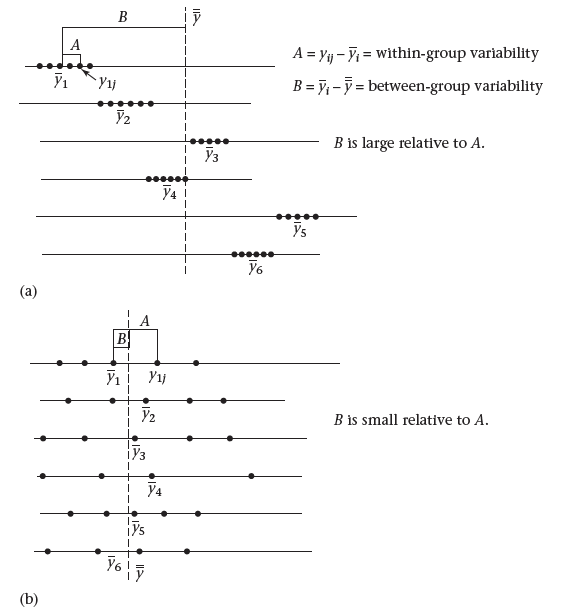其中组间平均平方和=组间MS=BetweenSS/(k-1)，k-1为组间自由度，组内平局平方和=组内MS=WithinSS/(n-k)，n-k为组内自由度，显著性检验建立在组间平均平方和与组内平均平方和的比值上，且该比值为F分布，自由度为k-1, n-k。 具体到R中，使用R中的PlantGrowth数据集举例。该数据有两个实验组和一个对照组，数据的整体情况上文已经统计过，下面通过box plot进行形象化展示（使用前文提到过的ggpubr包）。 xxxxxxxxxxlibrary(ggpubr)ggboxplot(PlantGrowth, x = "group", y = "weight", color = "group", palette = c("#2072b8", "#ff6a38", "#1e993b"), order = c("ctrl", "trt1", "trt2"), xlab = "Treatment", ylab = "Weight" )​ggline(PlantGrowth, x = "group", y = "weight", add = c("mean_se", "jitter"), order = c("ctrl", "trt1", "trt2"), ylab = "Weight", xlab = "Treatment")​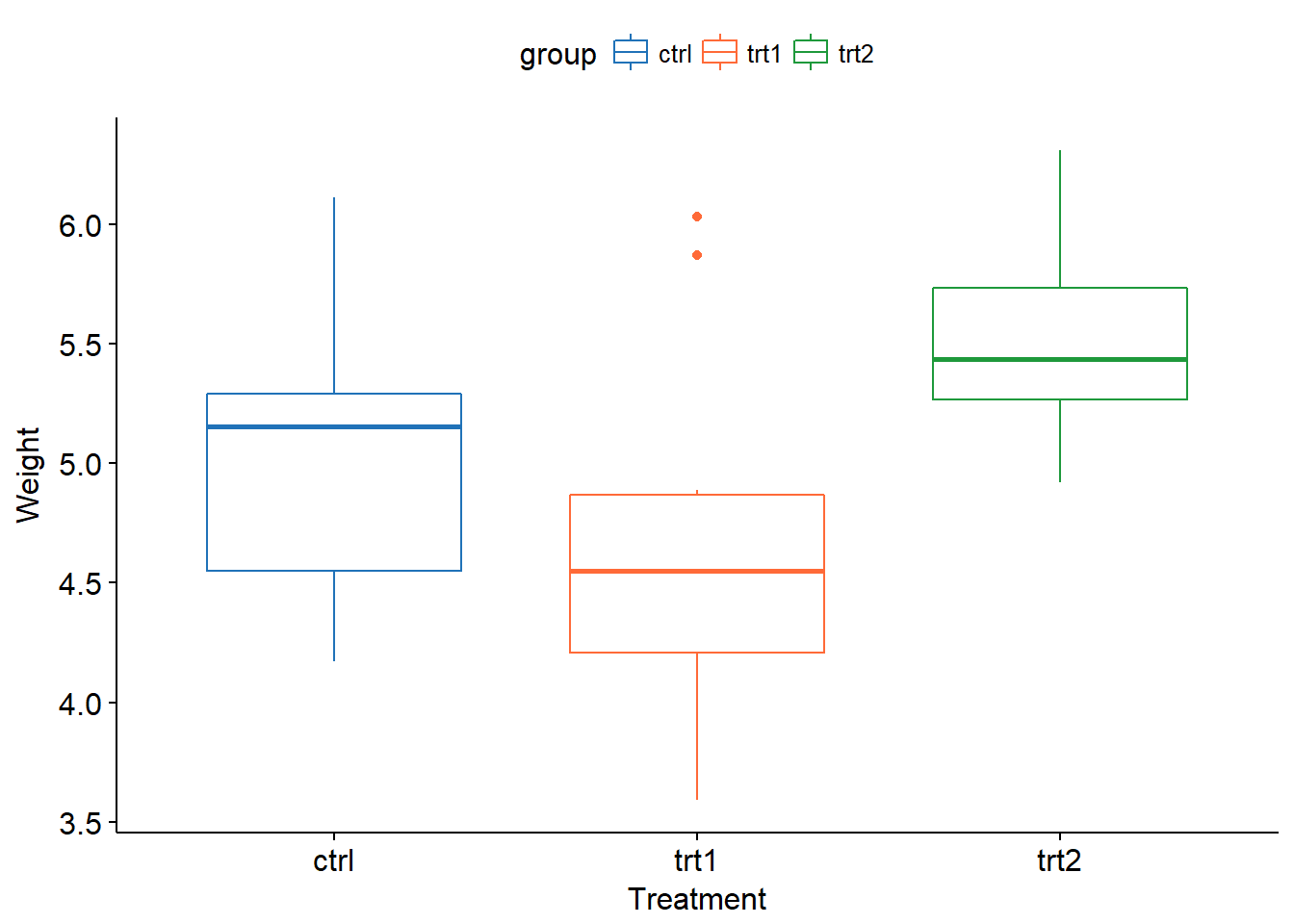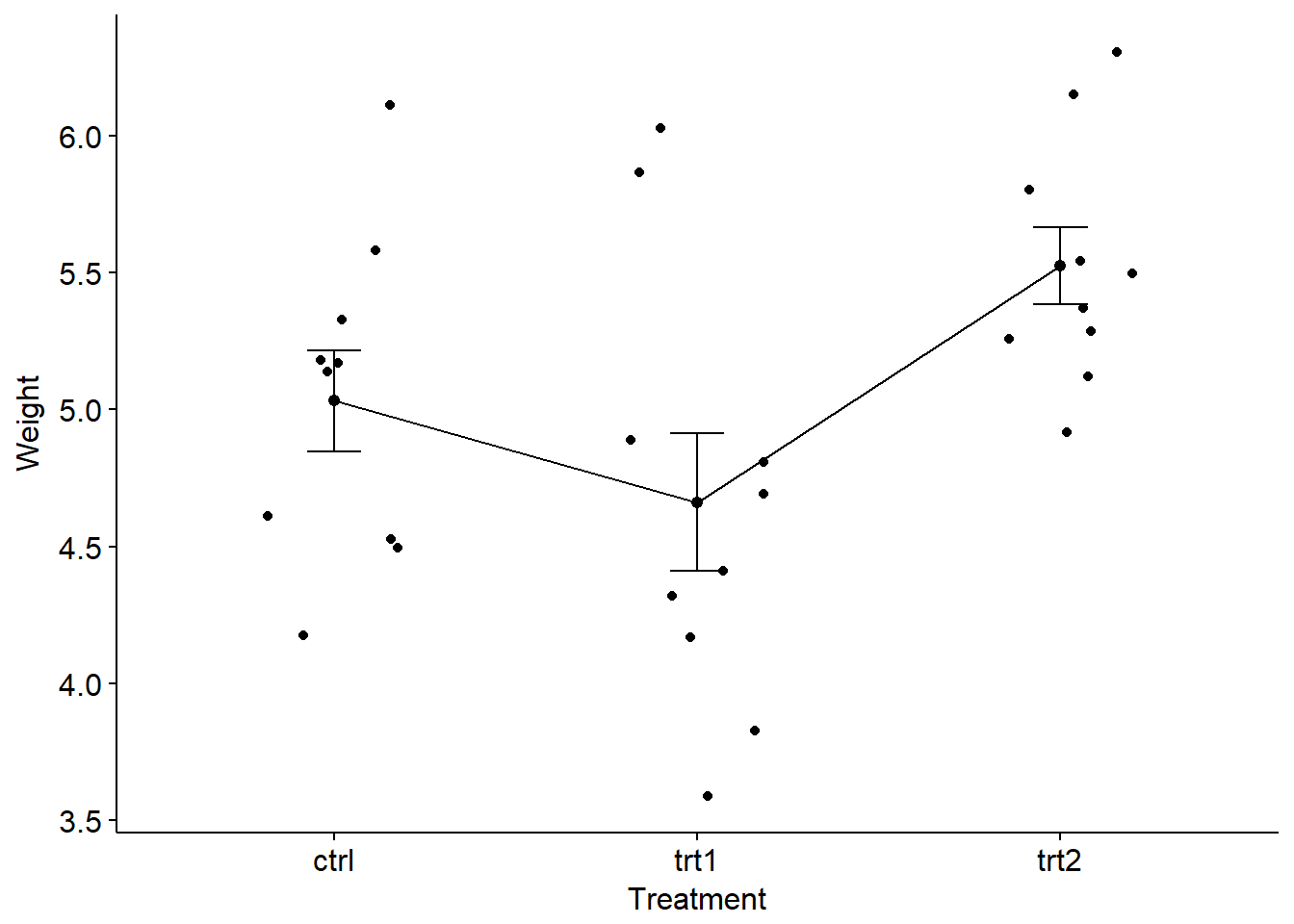检测正态分布和方差齐性 xxxxxxxxxx#检验方差齐性方法一bartlett.test(weight ~ group, data=PlantGrowth)​#检验方差齐性方法二library(car)leveneTest(PlantGrowth$weight~PlantGrowth$group)​# Bartlett test of homogeneity of variances## data: weight by group# Bartlett's K-squared = 2.8786, df = 2, p-value = 0.2371## Levene's Test for Homogeneity of Variance (center = median)# Df F value Pr(>F)# group 2 1.1192 0.3412# 27​ 不符合前提条件时 • 如果此步骤检测方差不齐性，可以使用Welch’s ANOVAoneway.test() • 如果不符合正态分布模型，可以使用非参数检验Kruskal-Wallis testkruskal.test()pairwise.wilcox.test() 进行one-way ANOVA 分析 xxxxxxxxxxpg.aov <- aov(weight ~ group, data = PlantGrowth)summary(pg.aov)​# Df Sum Sq Mean Sq F value Pr(>F)# group 2 3.766 1.8832 4.846 0.0159 *# Residuals 27 10.492 0.3886# ---# Signif. codes: 0 ‘***’ 0.001 ‘**’ 0.01 ‘*’ 0.05 ‘.’ 0.1 ‘ ’ 1​ 通过aov()，我们只能判断这三组数据之间均值不相等，但是不知道具体哪组存在差别。为了知道具体的差异情况，可以采用如下三种方法。 当进行任意两组间比较时，需要考虑由family-wise error rate 造成的p值误差，需要对p值进行校正以保证任何两组之间显著性差异的总体概率可以维持在一个固定的显著性水平。常用的校正方法"bonferroni"、"BH"和"fdr"等。 1. Pairewise t-test xxxxxxxxxxpairwise.t.test(PlantGrowth$weight, PlantGrowth$group, p.adjust.method = "fdr")​# Pairwise comparisons using t tests with pooled SD## data: PlantGrowth$weight and PlantGrowth$group## ctrl trt1# trt1 0.194 -# trt2 0.132 0.013## P value adjustment method: fdr 1. Tukey multiple pairwise-comparisons xxxxxxxxxxTukeyHSD(pg.aov)​# Tukey multiple comparisons of means# 95% family-wise confidence level## Fit: aov(formula = weight ~ group, data = PlantGrowth)##$group#             diff        lwr       upr     p adj# trt1-ctrl -0.371 -1.0622161 0.3202161 0.3908711# trt2-ctrl  0.494 -0.1972161 1.1852161 0.1979960# trt2-trt1  0.865  0.1737839 1.5562161 0.0120064​plot(TukeyHSD(pg.aov))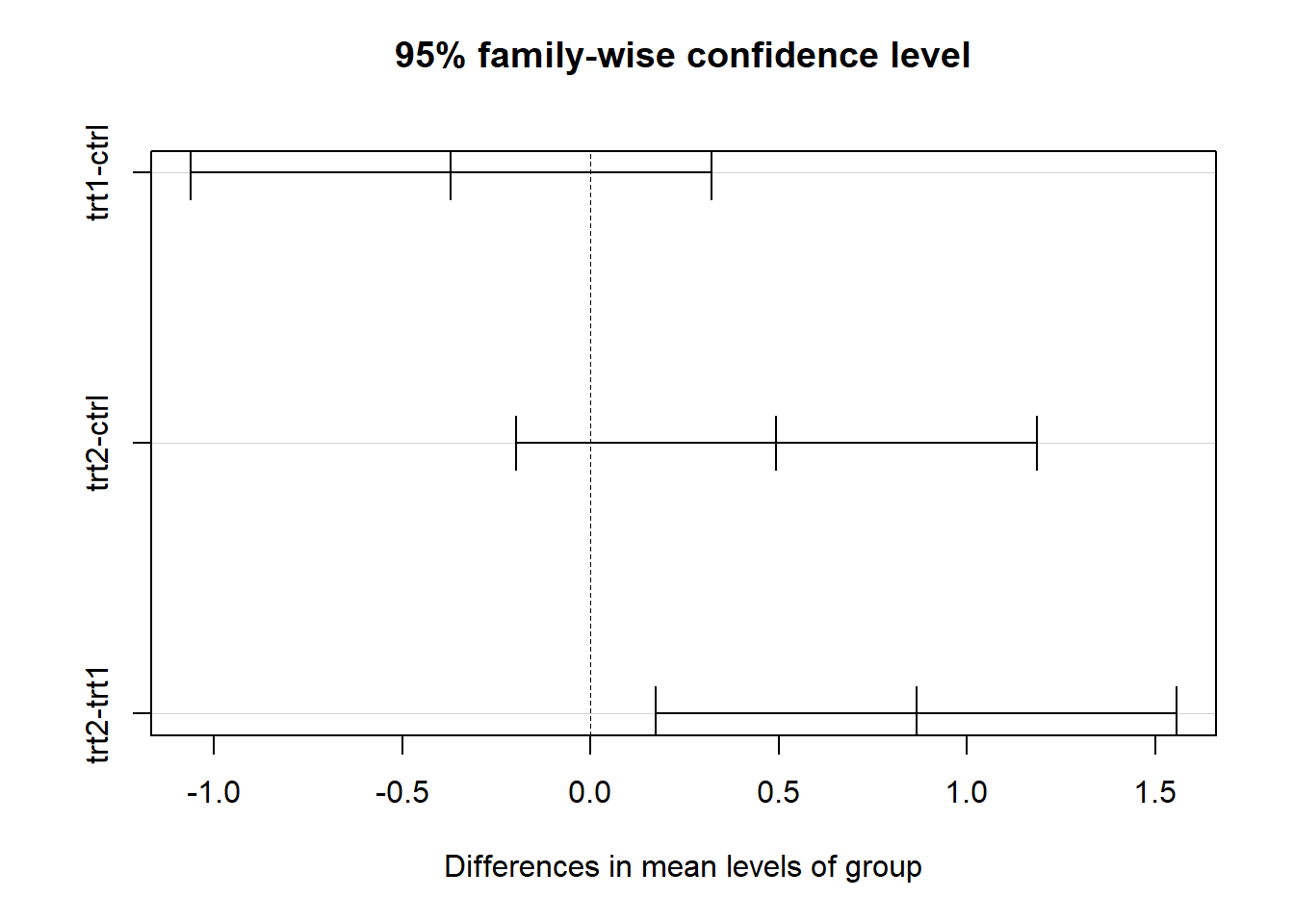1. multcomp包glht()
xxxxxxxxxxlibrary(multcomp)summary(glht(pg.aov, linfct=mcp(group="Dunnett")))summary(glht(pg.aov, linfct=mcp(group="Tukey")))​#    Simultaneous Tests for General Linear Hypotheses## Multiple Comparisons of Means: Dunnett Contrasts### Fit: aov(formula = weight ~ group, data = PlantGrowth)## Linear Hypotheses:#                  Estimate Std. Error t value Pr(>|t|)# trt1 - ctrl == 0  -0.3710     0.2788  -1.331    0.323# trt2 - ctrl == 0   0.4940     0.2788   1.772    0.153# (Adjusted p values reported -- single-step method)###    Simultaneous Tests for General Linear Hypotheses## Multiple Comparisons of Means: Tukey Contrasts### Fit: aov(formula = weight ~ group, data = PlantGrowth)## Linear Hypotheses:#                  Estimate Std. Error t value Pr(>|t|)# trt1 - ctrl == 0  -0.3710     0.2788  -1.331    0.391# trt2 - ctrl == 0   0.4940     0.2788   1.772    0.198# trt2 - trt1 == 0   0.8650     0.2788   3.103    0.012 *# ---# Signif. codes:  0 ‘***’ 0.001 ‘**’ 0.01 ‘*’ 0.05 ‘.’ 0.1 ‘ ’ 1# (Adjusted p values reported -- single-step method)#

### two-way ANOVA

xxxxxxxxxx# 检测不同条件样本量是否相等table(ToothGrowth$supp,ToothGrowth$dose)​#    0.5  1  2#  OJ  10 10 10#  VC  10 10 10​​summary(ToothGrowth)# 发现dose并没有当做因子来处理，需要进行转换​#      len        supp         dose# Min.   : 4.20   OJ:30   Min.   :0.500# 1st Qu.:13.07   VC:30   1st Qu.:0.500# Median :19.25           Median :1.000# Mean   :18.81           Mean   :1.167# 3rd Qu.:25.27           3rd Qu.:2.000# Max.   :33.90           Max.   :2.000​tg <- ToothGrowthtg$dose <- factor(tg$dose, levels = c(0.5,1,2),                  labels =c("low","mid","high") )# 重新检查summary(tg)​#      len        supp      dose# Min.   : 4.20   OJ:30   low :20# 1st Qu.:13.07   VC:30   mid :20# Median :19.25           high:20# Mean   :18.81# 3rd Qu.:25.27# Max.   :33.90​

xxxxxxxxxxsummary(tg.aov <- aov(tg$len~tg$supp*tg$dose))## 正确做法​# Df Sum Sq Mean Sq F value Pr(>F)# tg$supp          1  205.4   205.4  15.572 0.000231 ***# tg$dose 2 2426.4 1213.2 92.000 < 2e-16 ***# tg$supp:tg$dose 2 108.3 54.2 4.107 0.021860 *# Residuals 54 712.1 13.2# ---# Signif. codes: 0 ‘***’ 0.001 ‘**’ 0.01 ‘*’ 0.05 ‘.’ 0.1 ‘ ’ 1​summary(tmp.aov <- aov(ToothGrowth$len~ToothGrowth$supp*ToothGrowth$dose))## 错误做法，不将dose转换为factor，《R语言实战》第一版用到这个例子的时候书上是错误的，第二版已经改正。​#                                   Df Sum Sq Mean Sq F value   Pr(>F)# ToothGrowth$supp 1 205.4 205.4 12.317 0.000894 ***# ToothGrowth$dose                   1 2224.3  2224.3 133.415  < 2e-16 ***# ToothGrowth$supp:ToothGrowth$dose  1   88.9    88.9   5.333 0.024631 *# Residuals                         56  933.6    16.7# ---# Signif. codes:  0 ‘***’ 0.001 ‘**’ 0.01 ‘*’ 0.05 ‘.’ 0.1 ‘ ’ 1

xxxxxxxxxxlibrary(ggplot2)ggplot(tg, aes(x=dose,y=len))+geom_boxplot(aes(fill = dose))+facet_grid(.~supp)ggplot(tg, aes(x=supp,y=len))+geom_boxplot(aes(fill = supp))+facet_grid(.~dose)​library(ggpubr)ggboxplot(tg, x = "dose", y = "len", color = "supp",          palette = c("#2072b8", "#ff6a38"))ggboxplot(tg, x = "supp", y = "len", color = "dose",          palette = c("#2072b8", "#ff6a38", "#1e993b"))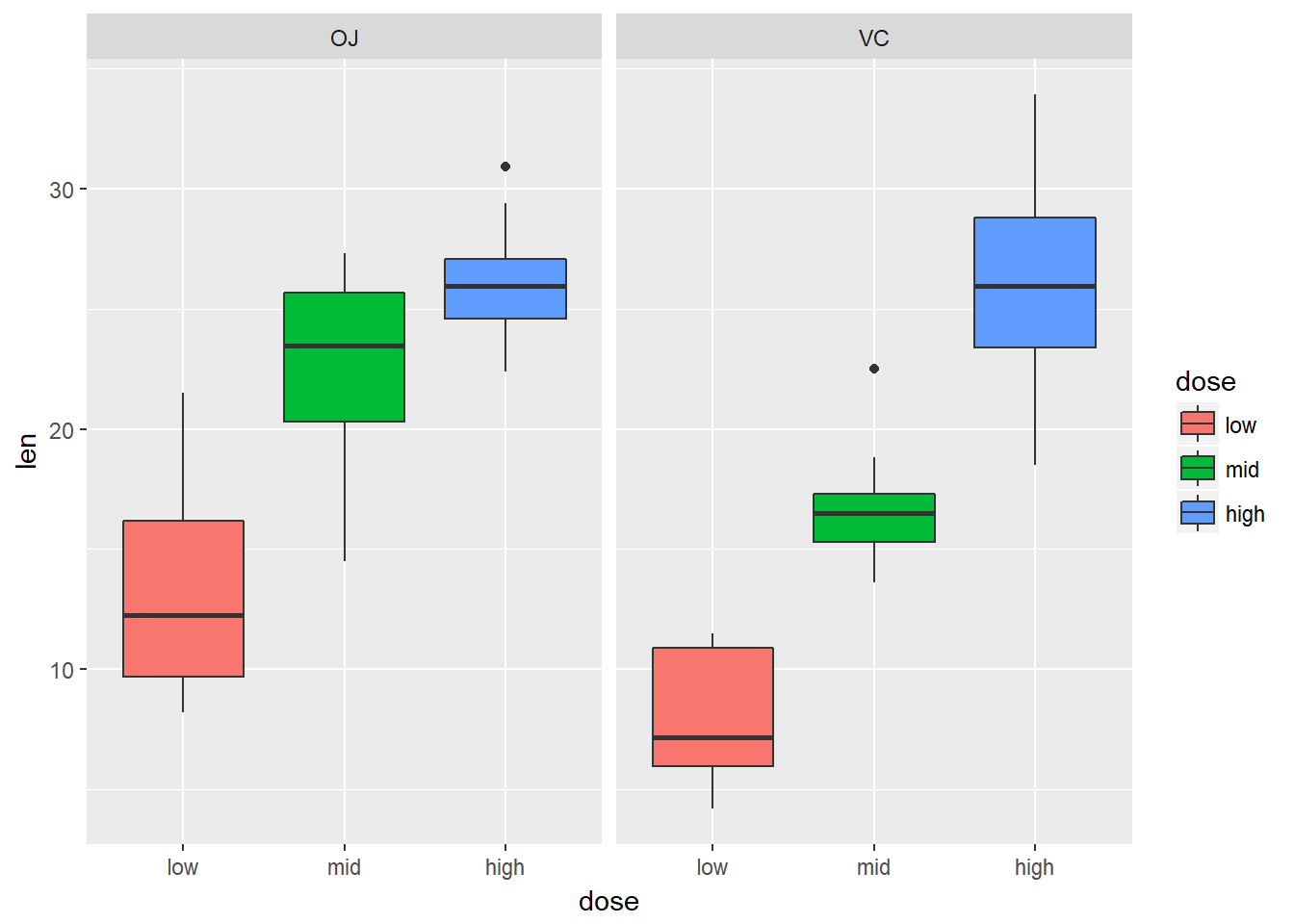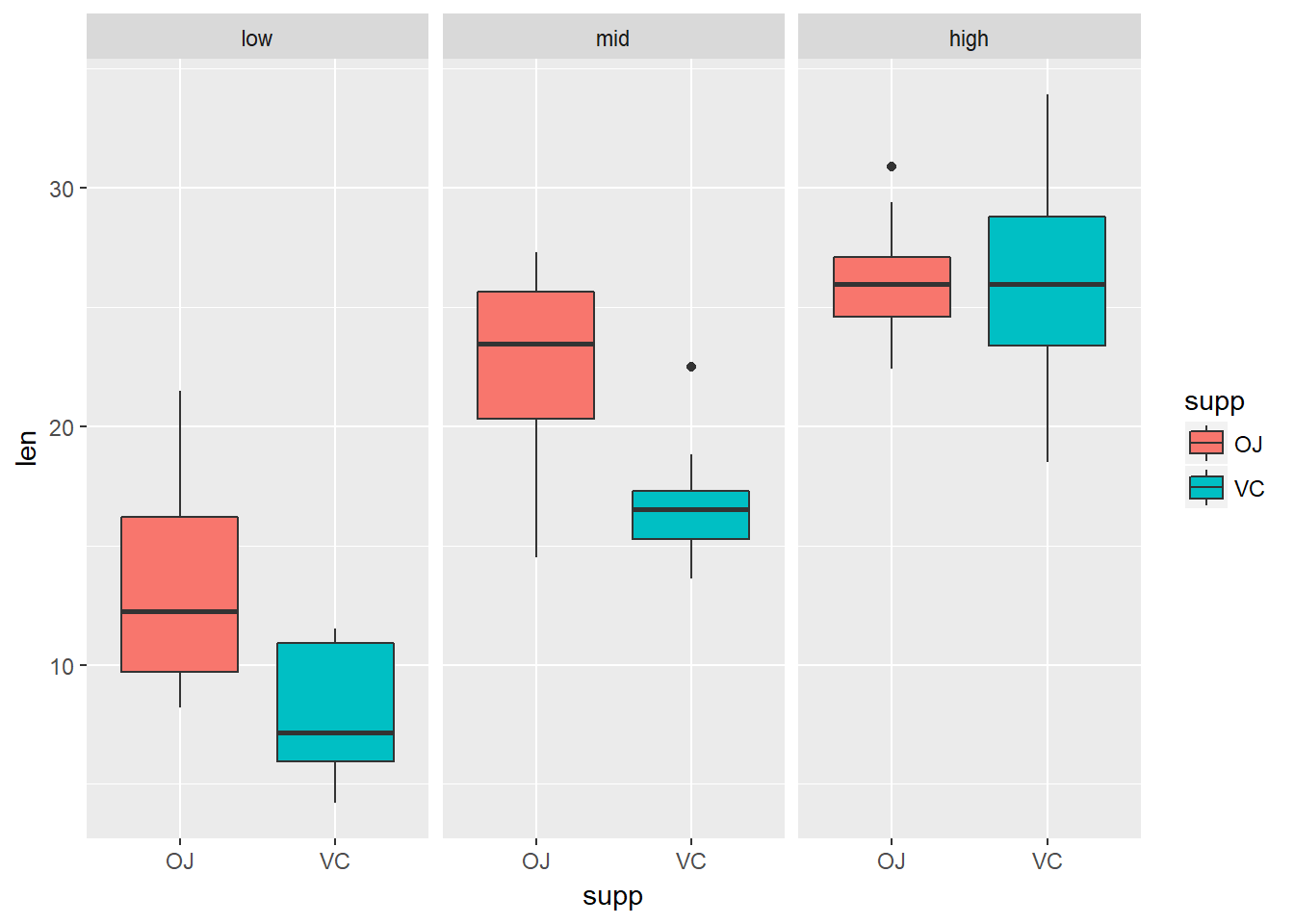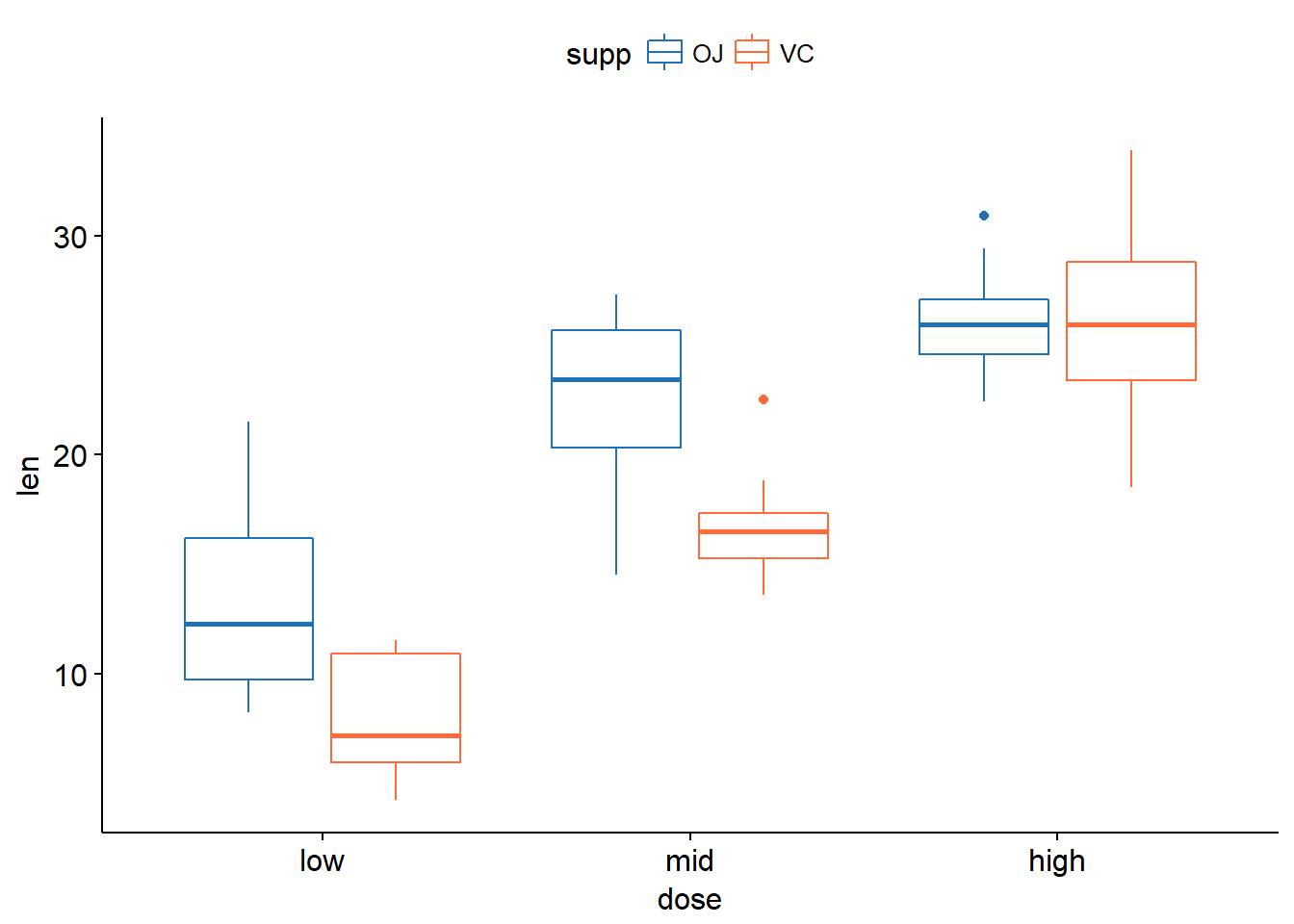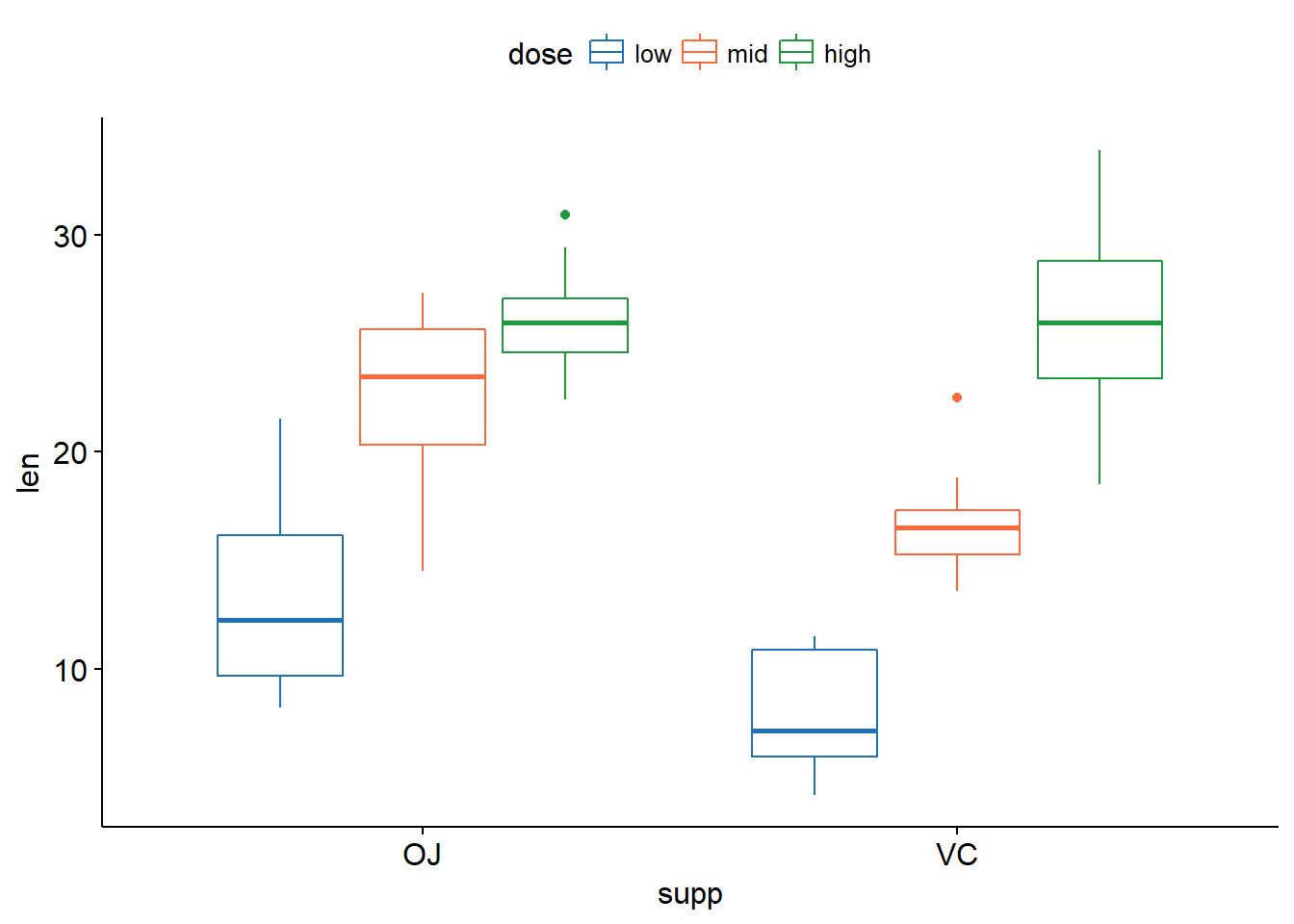xxxxxxxxxxTukeyHSD(tg.aov)​#   Tukey multiple comparisons of means#     95% family-wise confidence level## Fit: aov(formula = tg$len ~ tg$supp * tg$dose)##$tg$supp# diff lwr upr p adj# VC-OJ -3.7 -5.579828 -1.820172 0.0002312##$tg$dose# diff lwr upr p adj# mid-low 9.130 6.362488 11.897512 0.0e+00# high-low 15.495 12.727488 18.262512 0.0e+00# high-mid 6.365 3.597488 9.132512 2.7e-06##$tg$supp:tg$dose#                  diff        lwr        upr     p adj# VC:low-OJ:low   -5.25 -10.048124 -0.4518762 0.0242521# OJ:mid-OJ:low    9.47   4.671876 14.2681238 0.0000046# VC:mid-OJ:low    3.54  -1.258124  8.3381238 0.2640208# OJ:high-OJ:low  12.83   8.031876 17.6281238 0.0000000# VC:high-OJ:low  12.91   8.111876 17.7081238 0.0000000# OJ:mid-VC:low   14.72   9.921876 19.5181238 0.0000000# VC:mid-VC:low    8.79   3.991876 13.5881238 0.0000210# OJ:high-VC:low  18.08  13.281876 22.8781238 0.0000000# VC:high-VC:low  18.16  13.361876 22.9581238 0.0000000# VC:mid-OJ:mid   -5.93 -10.728124 -1.1318762 0.0073930# OJ:high-OJ:mid   3.36  -1.438124  8.1581238 0.3187361# VC:high-OJ:mid   3.44  -1.358124  8.2381238 0.2936430# OJ:high-VC:mid   9.29   4.491876 14.0881238 0.0000069# VC:high-VC:mid   9.37   4.571876 14.1681238 0.0000058# VC:high-OJ:high  0.08  -4.718124  4.8781238 1.0000000​#上述结果如果感觉过于复杂，可以使用下面下面的形式​TukeyHSD(tg.aov,which = "tg$dose")​# Tukey multiple comparisons of means# 95% family-wise confidence level## Fit: aov(formula = tg$len ~ tg$supp * tg$dose)## $tg$dose#            diff       lwr       upr   p adj# mid-low   9.130  6.362488 11.897512 0.0e+00# high-low 15.495 12.727488 18.262512 0.0e+00# high-mid  6.365  3.597488  9.132512 2.7e-06​# 或者pairwise.t.test(tg$len,tg$dose)​#   Pairwise comparisons using t tests with pooled SD## data:  tg$len and tg$dose##      low     mid# mid  1.3e-08 -# high 4.4e-16 1.4e-05## P value adjustment method: holm​

## 比例分析

### 单比例检验

$z = \frac{p_o-p_e}{\sqrt{p_oq/n}}$

xxxxxxxxxxprop.test(60, 100, p = 0.5, alternative = "greater")​#   1-sample proportions test with continuity# correction## data:  60 out of 100, null probability 0.5# X-squared = 3.61, df = 1, p-value = 0.02872# alternative hypothesis: true p is greater than 0.5# 95 percent confidence interval:#  0.5127842 1.0000000# sample estimates:#   p# 0.6

### 双比例检验

xxxxxxxxxx prop.test(x = c(400, 460), n = c(500, 500), alternative = "less")​#   2-sample test for equality of proportions with# continuity correction## data:  c(400, 460) out of c(500, 500)# X-squared = 28.912, df = 1, p-value = 3.787e-08# alternative hypothesis: less# 95 percent confidence interval:#  -1.0000000 -0.0824468# sample estimates:# prop 1 prop 2#   0.80   0.92

### 卡方分布

$\chi^2$分布可以通过原假设，得到一个统计量来表示期望结果和实际结果之间的偏离程度，进而根据分布，自由度和假设成立的情况，得出观察频率极值的发生概率（比当前统计结果更加极端的概率）。计算方法是对概率分布中的每一个频率，用期望频数和实际频数差的平方除以期望频数，最后把所有结果相加。得到的统计量结果越大，说明差别越显著，数值越小说明观察和期望的差别越小，当观察频数和期望频数一致是卡方为0。其实就是在比较观测到的比例和期望的比例的关系。

$\chi^2=\sum \frac{(O-E)^2}{E}$

## 常用高阶分析方法

### 聚类分析

#### Hierarchical Clustering

xxxxxxxxxx# 标准化usa_norm <- dist(scale(USArrests), method = "euclidean")​# 构建树hc <- hclust(usa_norm, method = "complete")​# install.packages(c("factoextra", "dendextend"))# 可视化展示library(factoextra)#基础plot(hc, hang = -1, cex = 0.7)​#美化fviz_dend(hc, k = 4,      # 分为4类          cex = 0.4,      # label大小，防止字不显示完全          k_colors = c("#2E9FDF", "#00AFBB", "#E7B800", "#FC4E07"),          color_labels_by_k = TRUE,  # color labels by groups          rect = TRUE, # Add rectangle around groups          rect_fill = TRUE,          rect_border = c("#2E9FDF", "#00AFBB", "#E7B800", "#FC4E07"),          ggtheme = theme_void() # ggplot2主题          )​# 环形fviz_dend(hc, cex = 0.4, k = 4,          k_colors = "jco", type = "circular")​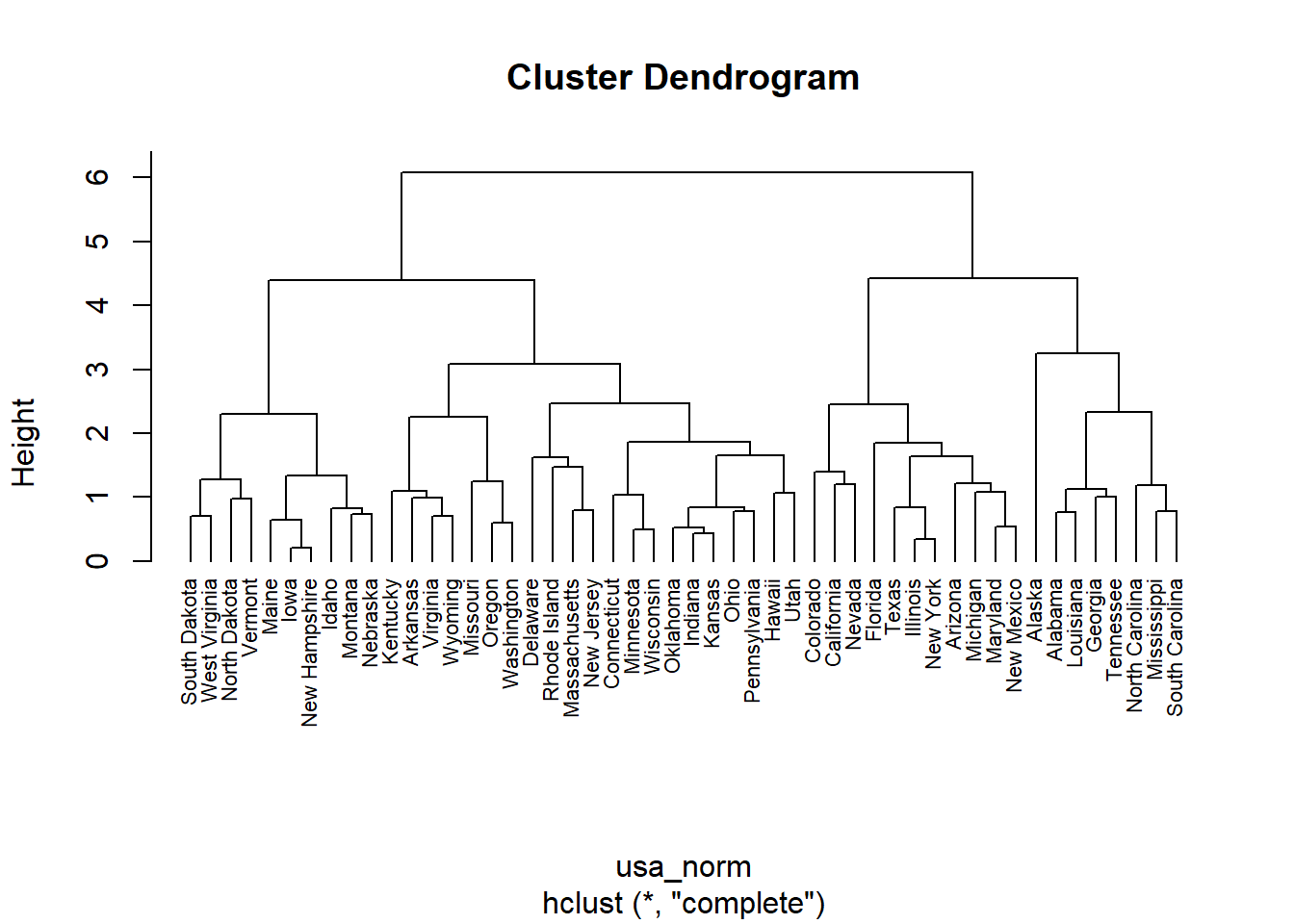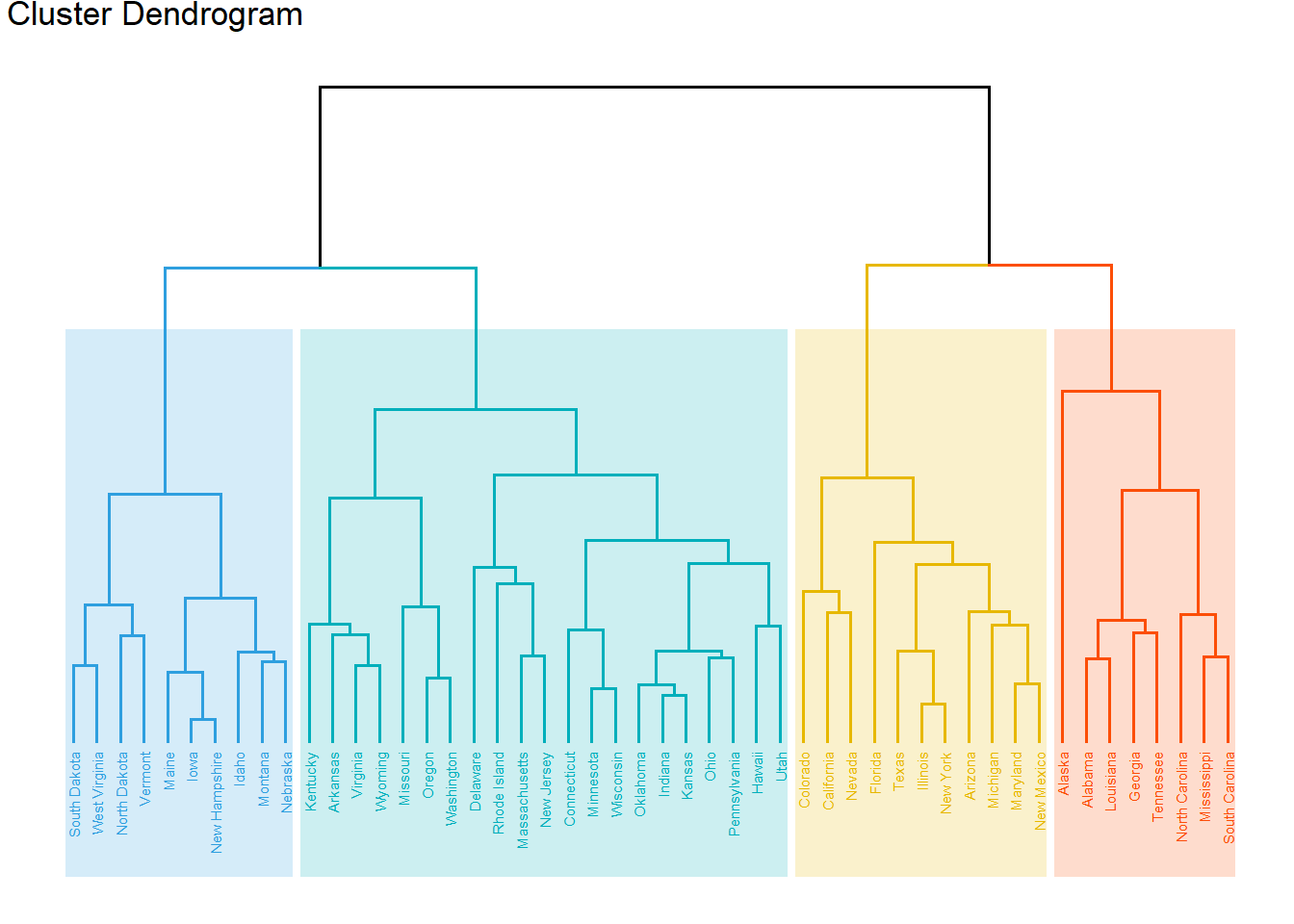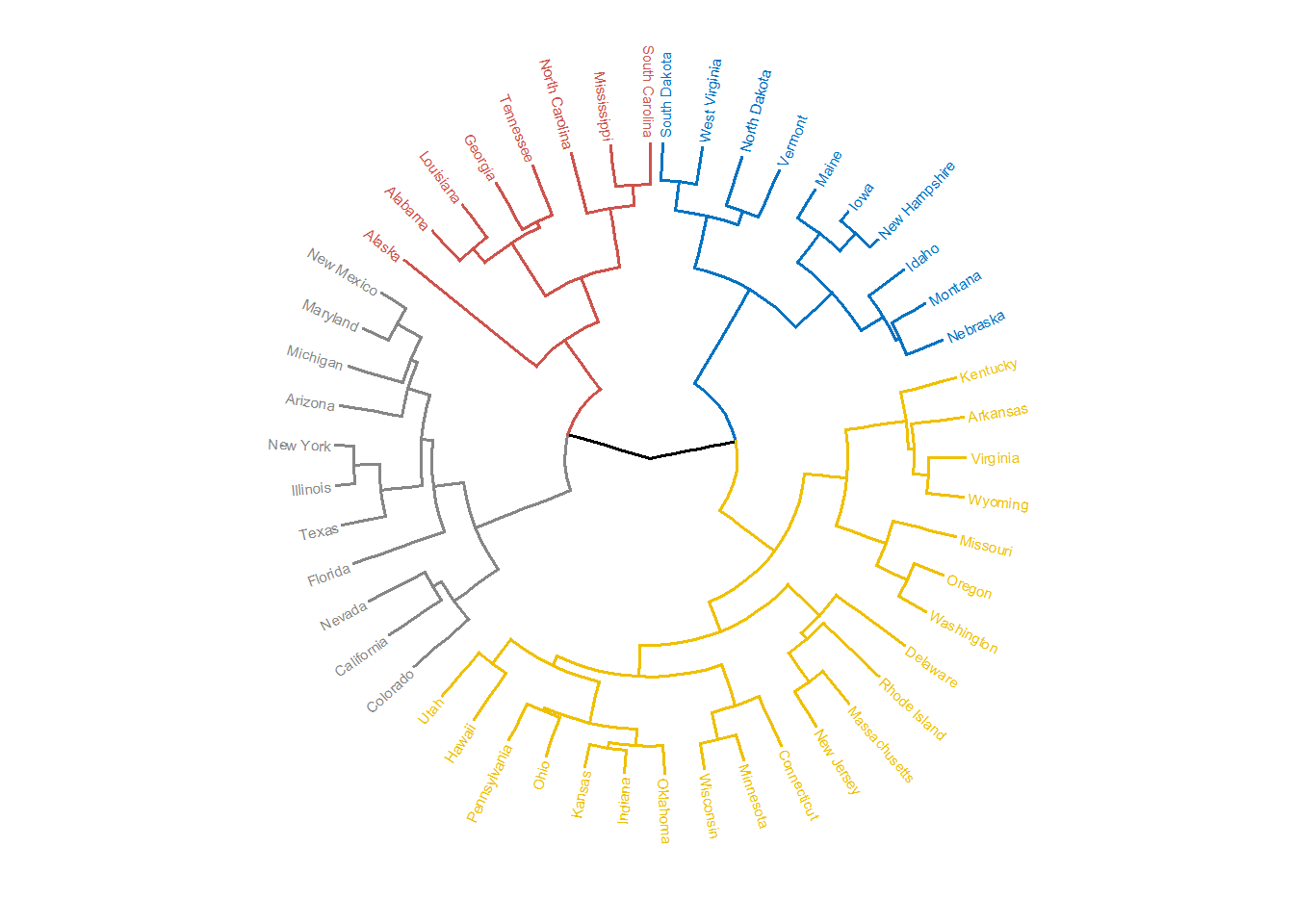### 主成分分析

xxxxxxxxxx# 只支持数值型变量，所以提取原数据集前4列ir_num <- iris[, 1:4]​# 进行pca分析ir_pca <- prcomp(ir_num,                 scale. = TRUE)# 查看主成分信息summary(ir_pca)​# Importance of components%s:#                           PC1    PC2     PC3     PC4# Standard deviation     1.7084 0.9560 0.38309 0.14393# Proportion of Variance 0.7296 0.2285 0.03669 0.00518# Cumulative Proportion  0.7296 0.9581 0.99482 1.00000​